﻿ 15组Excel函数，解决数据分析中80%的难题！ - 言言说历史# 15组Excel函数，解决数据分析中80%的难题！

01 IF函数

=IF(判断条件,条件成立返回的值,条件不成立返回的值）

=IF(AND(条件1,条件2), 两个条件同时成立返回的值,不成立返回的值)

=IF(OR(条件1,条件2), 两个条件之一成立返回的值,都不成立返回的值)

=IF(AND(A2<500,B2="未到期"),"补款",””)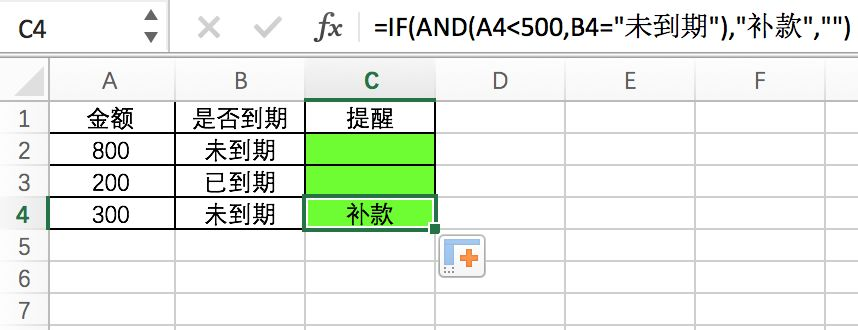02 Round函数、INT函数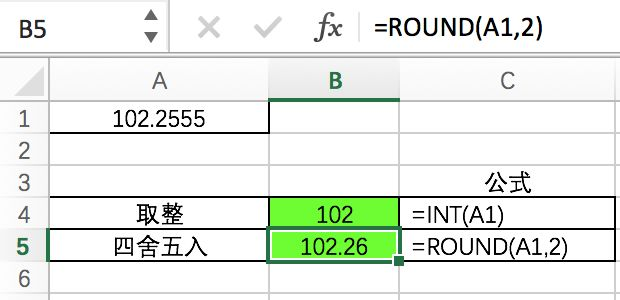03 Vlookup函数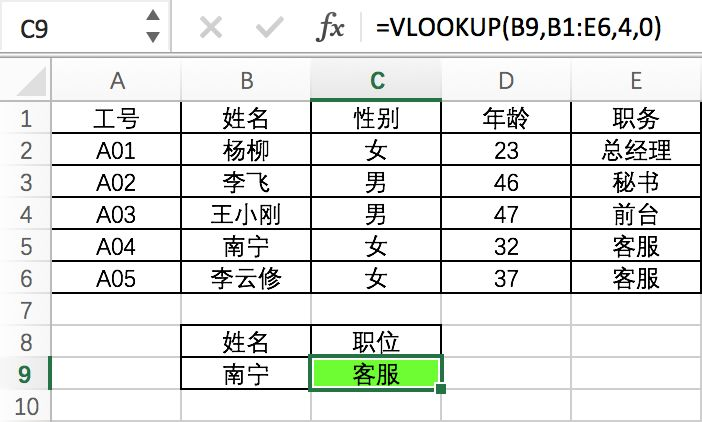04 Sumif和Countif函数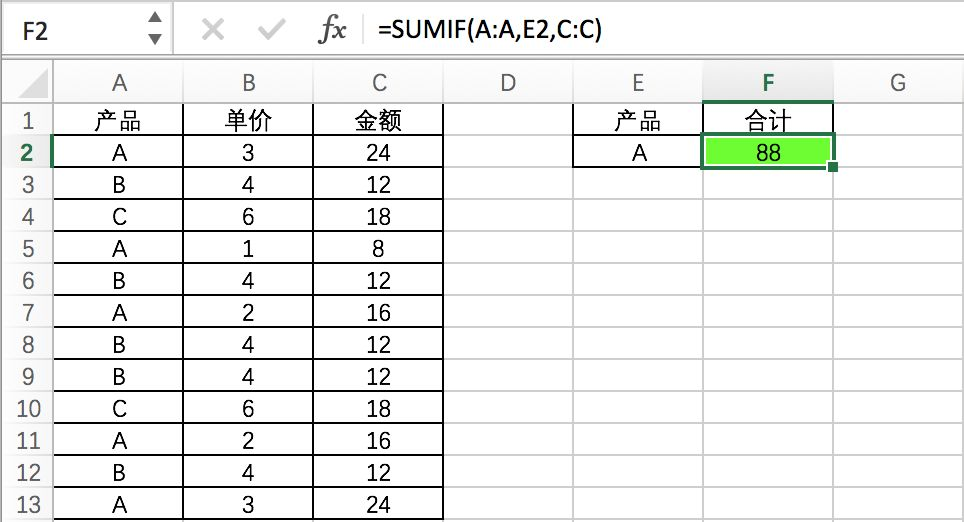05 Sumifs和Countifs函数

=Sumifs(求和区域,判断区域1，条件1，判断区域2，条件2…..

=Countifs(判断区域1，条件1，判断区域2，条件2.....）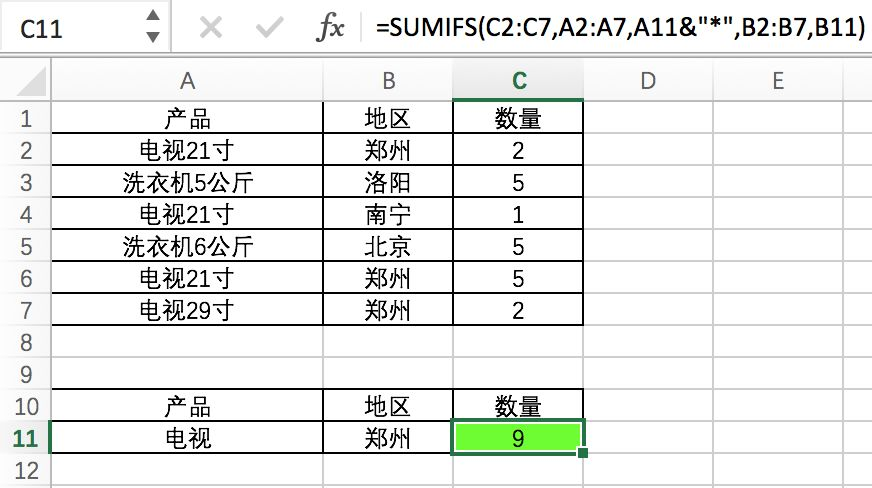06 Left、Right和Mid函数

=Left(字符串,从左边截取的位数)

=Right(字符串,从右边截取的位数)

=Mid(字符串,从第几位开始截,截多少个字符）

=left("abcde",2)  结果为  ab

=right("abcde",3) 结果为 cde

=mid("abcde", 2,3) 结果为 bcd

07 Datedif函数

=Datedif(开始日期,结束日期."y") 间隔的年数

=Datedif(开始日期,结束日期."M") 间隔的月份

=Datedif(开始日期,结束日期."D") 间隔的天数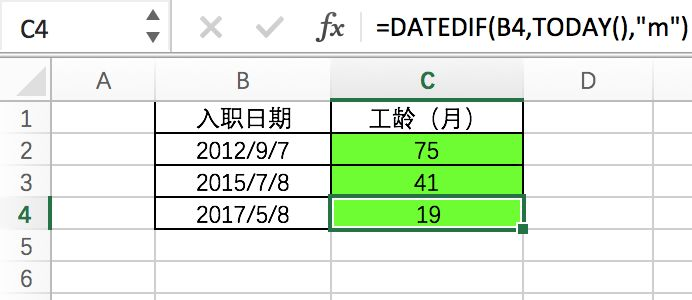08 最值计算函数

=MAX(区域) 返回最大值

=MIN(区域) 返回最小值

=Large(区域,n）返回第n大值

=Small(区域,n）返回第n小值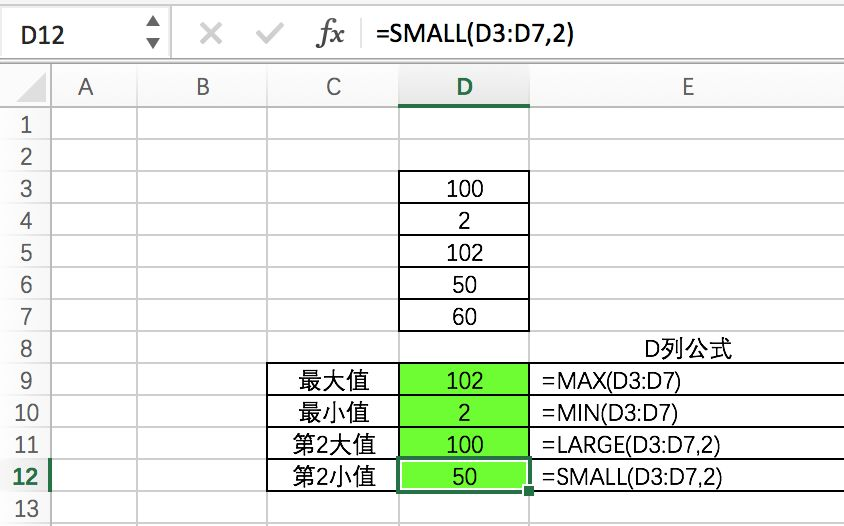09 IFERROR函数

=IFERROR(公式表达式,错误值转换后的值）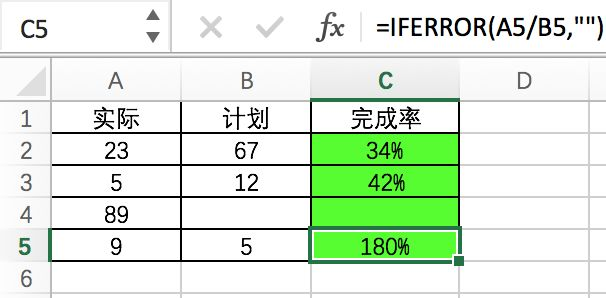10 INDEX+MATCH函数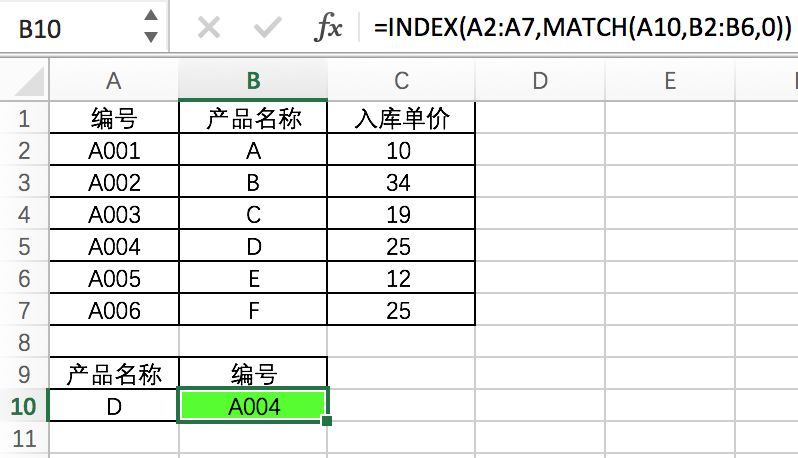11 FREQUENCY函数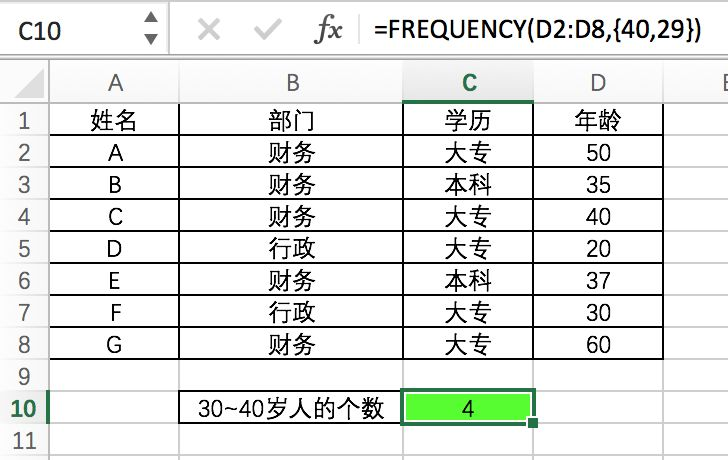12 AVERAGEIFS函数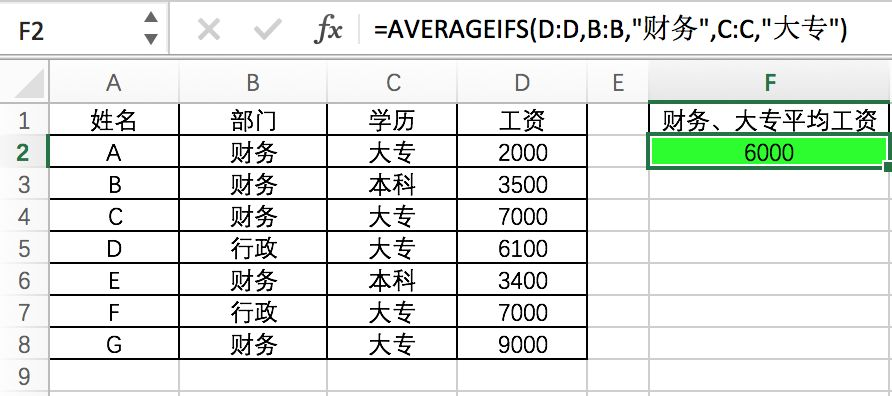13 SUMPRODUCT函数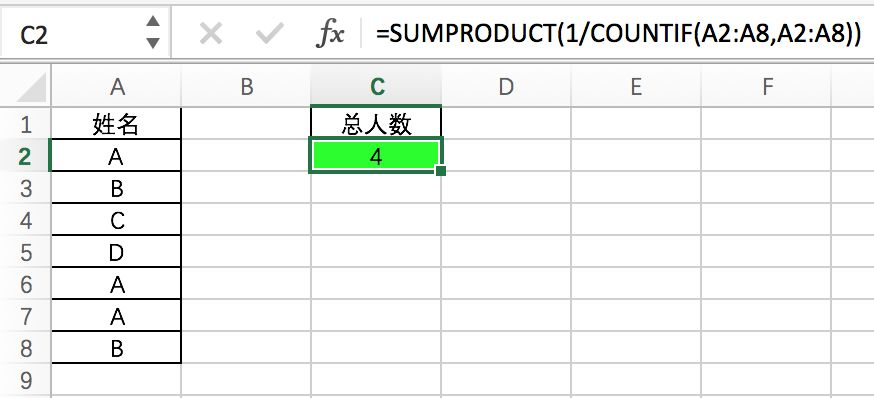14 PHONETIC函数

Phonetic函数只能对字符型内容合并，数字不可以。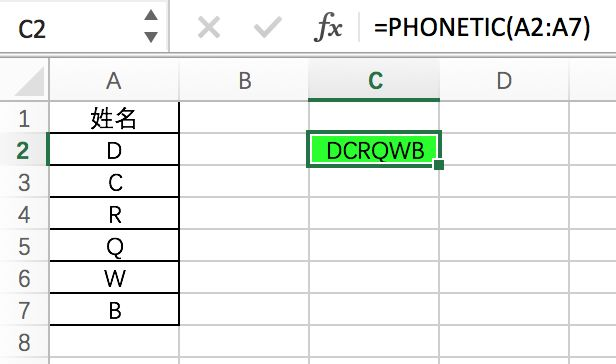15 SUBSTITUTE函数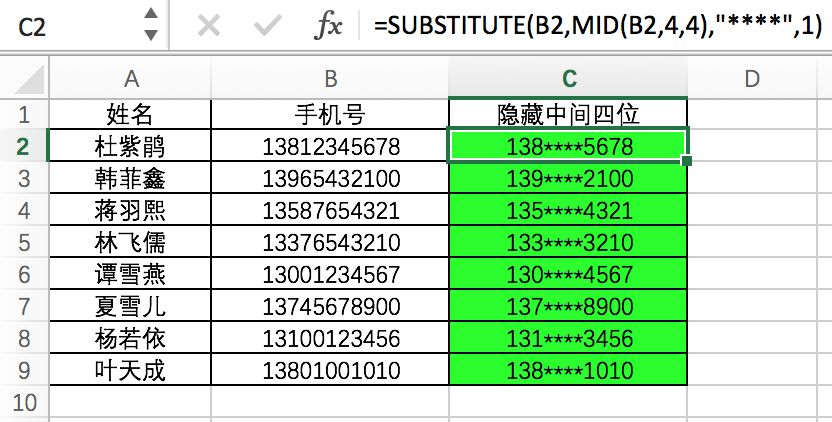-END-【网舟科技】